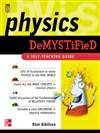# Physics Demystified: A Self-Teaching Guide

YOU DON'T HAVE TO BE A ROCKET SCIENTIST TO UNDERSTAND PHYSICS

Now anyone with an interest in the physical sciences can master physics -- without formal training or drowning in a sea of complicated formulas and equations. In Physics Demystified best-selling author Stan Gibilisco offers a fun, effective, and totally painless way to learn the fundamentals and general concepts of physics.

With Physics Demystified you master the subject one simple step at a time – at your own speed. Unlike most books on physics, general principles are presented first – and the details follow. In order to make the learning process as clear and simple as possible, heavy-duty math, formulas, and equations are kept to a minimum. This unique self-teaching guide offers questions at the end of each chapter and section to pinpoint weaknesses, and a 100-question final exam to reinforce the entire book.

Simple enough for a beginner but challenging enough for an advanced student, Physics Demystified is your direct route to learning or brushing up on physics.

HERE'S EVERYTHING YOU NEED TO:
* Understand the math used in physical science
* Solve mass/force/acceleration problems
* Create mathematical models of physical phenomena
* Perform distance vs. time calculations
* Determine potential and kinetic energy
* Calculate the wavelength of sounds and radio signals
* Understand visible light interference patterns
* Calculate the energy and frequency of a moving particle
* Understand atomic structure
* Learn about electric current, voltage, resistance, power, and energy

# 下载地址

• 本书版权归原作者所有，本站暂无下载，请购买正版！
•